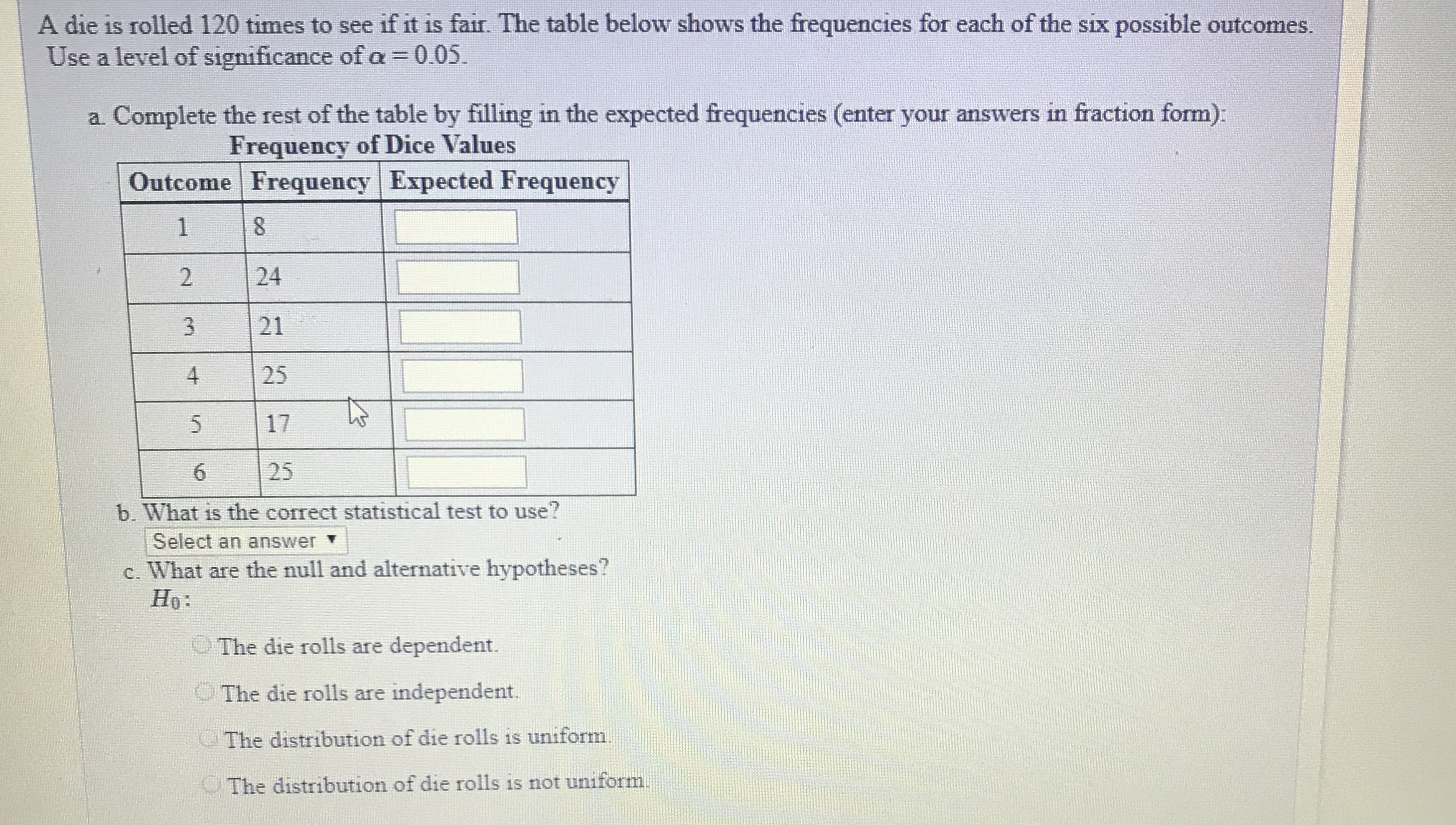A die is rolled 120 times to see if it is fair. The table below shows the frequencies for each of the six possible outcomes.Use a level of significance of α-0.05.a. Complete the rest of the table by filling in the expected frequencies (enter your answers in fraction form)Frequency of Dice ValuesOutcome Frequency Expected Frequency2 243 214 25172526b. What is the correct statistical test to use?Select an answer Tc. What are the null and alternative hypotheses?Ho:The die rolls are dependent.The die rolls are independent.The distribution of die rolls is uniformThe distribution of die rolls is not uniform

Questionhelp_outlineImage TranscriptioncloseA die is rolled 120 times to see if it is fair. The table below shows the frequencies for each of the six possible outcomes. Use a level of significance of α-0.05. a. Complete the rest of the table by filling in the expected frequencies (enter your answers in fraction form) Frequency of Dice Values Outcome Frequency Expected Frequency 2 24 3 21 4 25 17 25 2 6 b. What is the correct statistical test to use? Select an answer T c. What are the null and alternative hypotheses? Ho: The die rolls are dependent. The die rolls are independent. The distribution of die rolls is uniform The distribution of die rolls is not uniform fullscreen
Step 1

Given that a dice is rolled 120 times. Here we need to check if it is a fair die. In fair die the probability of occurrence of each number will be same = 1/6.

Using this we calculate the expected frquencies for outcomes as shown below

Step 2

Here we are testing for dice to be fair and we calclated expected frequencies and using the given observed freq...

Want to see the full answer?

See Solution

Want to see this answer and more?

Our solutions are written by experts, many with advanced degrees, and available 24/7

See Solution
Tagged in

Hypothesis Testing